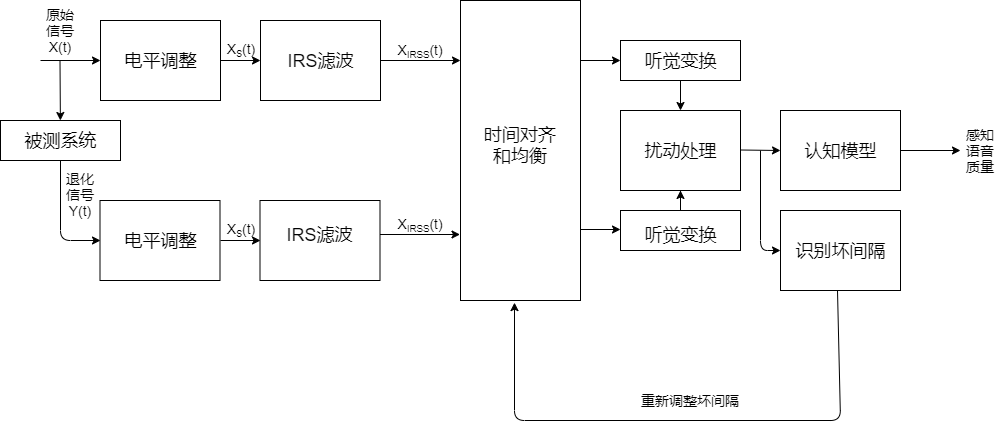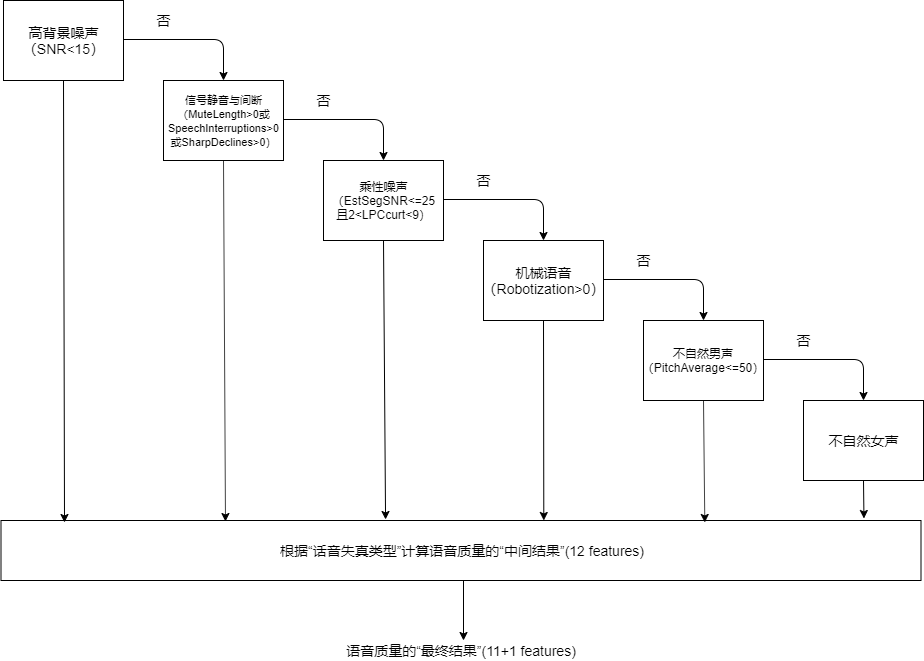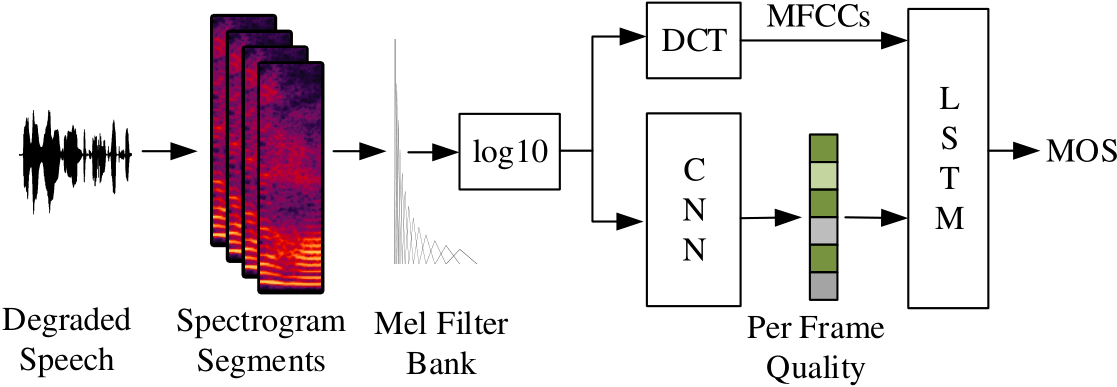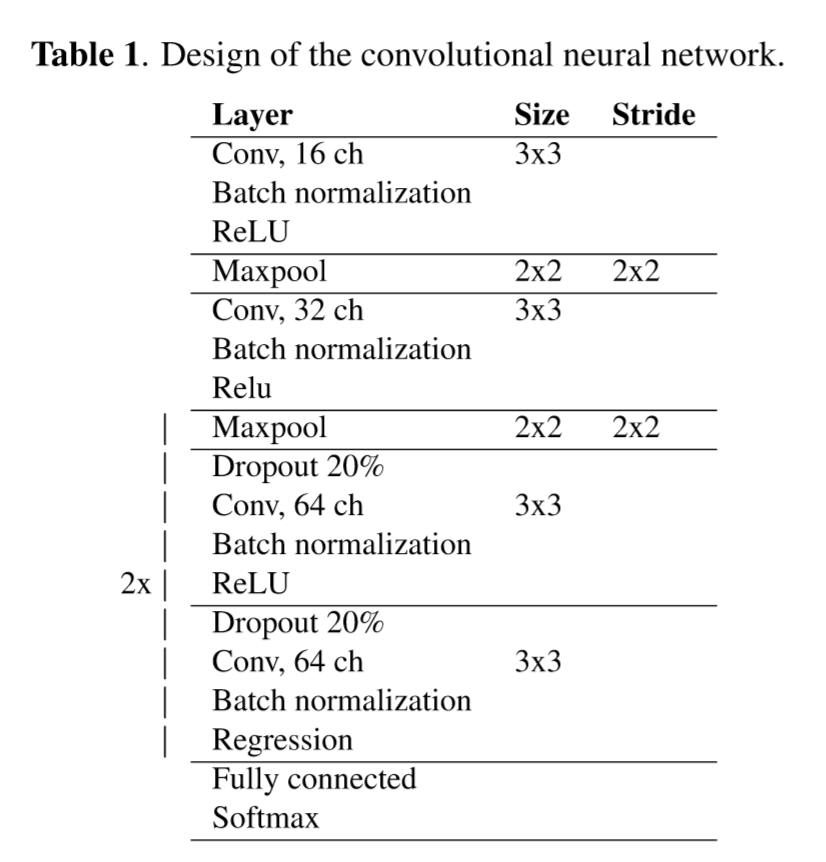# 语音质量评估

### 语音质量评测方法

• 主观评价：MOS, CMOS, ABX Test

• 客观评价

• 有参考质量评估(intrusive method)：ITU-T P.861(MNB), ITU-T P.862(PESQ), ITU-T P.863(POLQA), STOI, BSSEval
• 无参考质量评估(non-intrusive method)
• 传统方法
• 基于信号：ITU-T P.563, ANIQUE+
• 基于参数：ITU-T G.107(E-Model)
• 基于深度学习的方法：AutoMOS, QualityNet, NISQA, MOSNet

 An algorithm for predicting the intelligibility of speech masked by An algorithm for predicting the intelligibility of speech masked by modulated noise maskers

 Latent Variable Analysis and Signal Separation: 14th International Conference, LVA/ICA 2018, Surrey, UK

 Kim D S. ANIQUE: An auditory model for single-ended speech quality estimation[J]. IEEE Transactions on Speech and Audio Processing, 2005, 13(5): 821-831.

 AutoMOS

 QualityNet

 MOSNet

 G. Mittag and S. Möller, "Non-intrusive Speech Quality Assessment for Super-wideband Speech Communication Networks,"ICASSP 2019 - 2019 IEEE International Conference on Acoustics, Speech and Signal Processing (ICASSP), Brighton, United Kingdom, 2019, pp. 7125-7129.

### 平均意见得分(Mean Opnion Score, MOS)主观语音评测

MOS评测实际是一种很宽泛的说法。由于给出评测分数的是人类，因此可以灵活的测试语音的不同方面。比如在语音合成领域，常见的有自然度MOS(MOS of naturalness)，相似度MOS(MOS of similarity)。在实时通讯领域，有收听质量(Listening Quality)评价和对话质量(Conversational Quality)评价。但是人类给出的评分结果受到的干扰因素特别多，一般不同论文给出的MOS不具有非常明确的可比性，同一篇文章中的MOS才可以比较不同系统的优劣。谷歌在SSW10发表的Evaluating Long-form Text-to-Speech: Comparing the Ratings of Sentences and Paragraphs对若干种多行文本合成语音的评估方法进行了比较，在评估较长文本中的单个句子时，音频样本的呈现形式会显著影响被测人员给出的结果。比如仅提供单个句子而不提供上下文，与相同句子给出语境相比，被测人员给出的评分结果差异显著。

4.0~5.0 很好，听得清楚；延迟小，交流流畅
3.5~4.0 稍差，听得清楚；延迟小，交流欠流畅，有点杂音
3.0~3.5 还可以，听不太清；有一定延迟，可以交流
1.5~3.0 勉强，听不太清；延迟较大，交流需要重复多遍
0~1.5 极差，听不懂；延迟大，交流不通畅

MOS测试一般要求：

1. 足够多样化的样本（即试听者和句子数量）以确保结果在统计上的显著；

2. 控制每个试听者的实验环境和设备保持一致；

3. 每个试听者遵循同样的评估标准。

# -*- coding: utf-8 -*-
# @Time    : 2019/4/30 13:56
# @Author  : MengnanChen
# @FileName: caculate_MOS.py
# @Software: PyCharm

import math
import numpy as np
import pandas as pd
from scipy.linalg import solve
from scipy.stats import t

def calc_mos(data_path: str):
'''
计算MOS，数据格式：MxN，M个句子，N个试听人，data_path为MOS得分文件，内容都是数字，为每个试听的得分
:param data_path:
:return:
'''
mu = np.mean(data.values)
var_uw = (data.std(axis=1) ** 2).mean()
var_su = (data.std(axis=0) ** 2).mean()
mos_data = np.asarray([x for x in data.values.flatten() if not math.isnan(x)])
var_swu = mos_data.std() ** 2

x = np.asarray([[0, 1, 1], [1, 0, 1], [1, 1, 1]])
y = np.asarray([var_uw, var_su, var_swu])
[var_s, var_w, var_u] = solve(x, y)
M = min(data.count(axis=0))
N = min(data.count(axis=1))
var_mu = var_s / M + var_w / N + var_u / (M * N)
df = min(M, N) - 1  # 可以不减1
t_interval = t.ppf(0.975, df, loc=0, scale=1)  # t分布的97.5%置信区间临界值
interval = t_interval * np.sqrt(var_mu)
print('{} 的MOS95%的置信区间为：{} +—{} '.format(data_path, round(float(mu), 3), round(interval, 3)))

if __name__ == '__main__':
data_path = ''
calc_mos(data_path)


### 语音质量的感知评估(Perceptual evaluation of speech quality, PESQ)

PESQ在国际电信联盟的标注化代号为ITU-T P.862。总的想法是：

1. 对原始信号和通过被测系统的信号首先电平调整到标准听觉电平，再利用IRS（Intermediate Reference System）滤波器模拟标准电话听筒进行滤波；
2. 对通过电平调整和滤波之后的两个信号在时间上对准，并进行听觉变换，这个变换包括对系统中线性滤波和增益变化的补偿和均衡；
3. 将两个听觉变换后的信号之间的谱失真测度作为扰动（即差值），分析扰动曲面提取出的两个退化参数，在频率和时间上累积起来，映射到MOS的预测值。#### 电平调整和时间对齐

• 电平调整

由于各个系统的增益不同，因此需要将原信号和被测信号二者调整到统一、恒定的响度。

$X(t)$是原始信号的时域表示，而$Y(t)$$X(t)$通过被测系统后的输出信号，两者首先进行电平调整，将其统一到76 dB SPL(Sound Pressure Level)的能量水平。电平调整的流程：

• 首先对参考信号$X(t)$和退化信号$Y(t)$进行带通滤波：低于250Hz直接被过滤掉，在250Hz~2kHz之间不进行操作，高于2kHz采取衰减规则。
• 计算全局电平调整因子。如最大最小归一化，或者标准归一化参考信号和退化信号，将其调整到同一能量级上。电平调整后输出$X_s(t)$$Y_s(t)$
• IRS滤波

听觉测试需要使用IRS(Intermeiate Reference System, 中间参考系统)滤波来对参考语音信号和退化信号进行滤波，这种滤波主要是模拟典型的电话手柄听筒的频率响应特性。仿IRS滤波的实现过程为：

• 对整个语音文件进行FFT计算；
• 在频域，由具有与IRS接收滤波器相似的分段线性相应的滤波器滤波；
• 对语音文件进行FFT反变换。

通过IRS滤波系统后得到$X_{IRSS}(t)$$Y_{IRSS}(t)$.

#### 时间对齐

听觉变换时利用快速傅里叶变换逐帧进行频谱计算，然后再映射到感觉频域和响度，计算PESQ得分需要的参数也是逐帧计算得到的。PESQ算法的时间对齐是对在原始输入信号和退化输出语音之间的一系列延时进行计算，每一个时间间隔内的延时与前一个具有明显不同的时延值。在每一个时间间隔内，计算相应的起点和终点之间的时延，时间校准的原理就是，假设在同一个时间间隔内，原始语音和退化语音在相应的间隔内应该具有确定的时延值。PESQ算法采用了一种新的时间对齐算法，这种算法是基于包络的延迟估计方法。

### 感知模型

感知模型在PESQ算法中用来计算PESQ得分，即原始信号和退化信号之间的差异。它是一个心理模型，能够对客观语音质量评估提供一个主观MOS的预测值。PESQ可以映射到MOS刻度范围，计算一般分为：听觉变换，计算干扰密度，计算不对称因子，扰动聚合，坏间隔重对齐，最终计算获得PESQ得分。对于正规的主观测试，得分在1.0~4.5之间。在退化严重时，得分可能会低于1.0，但这种情况很少见。

### 客观评测算法的有效性

语音质量客观评价方法的性能优劣，一般用语音质量的客观MOS值和主观MOS值之间的相关程度和绝对误差作为评价的性能指标。两者的相关程度可以采用Pearson系数加以描述：

$\rho=\frac{\sum_{i=1}^N(MOS_o(i)-\overline{MOS_o})(MOS_s(i)-\overline{MOS_s})}{\sqrt{\sum_{i=1}^N(MOS_o(i)-\overline{MOS_o})^2\sum_{i=1}^N(MOS_s(i)-\overline{MOS_s})^2}}$

其中，$N$为样本数，$MOS_s(i)$是第$i$个样本人类评判的MOS分值，$MOS_o(i)$是第$i$个样本用客观评估方法预测出的客观MOS值。$\overline{MOS_s}$$\overline{MOS_o}$为两者的算术平均值。相关性系数描述了主客观评价的线性相关程度，相关系数越接近+1，客观评测算法越准确。绝对误差为：

$e_n=|MOS_o(i)-MOS_s(i)|$

其中，$MOS_s(i)$是第$i$个样本人类评判的MOS值，$MOS_o(i)$是第$i$个样本用客观评估方法预测出的客观MOS值。

### 客观语音质量评估的单端方法P.563

P.563和PESQ最大的区别就是，P.563只需要经过音频引擎传输后的输出信号，不需要原始信号，直接可以输出该信号的流畅度。因此，P.563的可用性更高，但是其准确性要比PESQ低。

P.563算法主要由三个部分组成：

• 预处理；
• 特征参数估计；
• 感知映射模型

P.563将语音进行预处理后，首先计算出若干个最重要的特征参数，根据这些特征参数判断语音的失真类型，失真类型直接决定了感知映射模型的系数和所使用的特征。利用感知映射模型（其实就是线性方程）计算得到最终的评价结果。

#### 预处理

$threshold=\overline{x_{noise}}-x\times \sqrt{\sum_{n=1}^N\frac{(x_{noise}(n)-\overline{x_{noise}})^2}{N}}$

$\overline{x_{noise}}=\sum_{n=1}^N\frac{x_{noise}(n)}{N}$

#### 特征参数提取

P.563算法在特征提取过程中利用人类的发声和听觉感知原理，不需要考虑通讯网络相关的信息。P.563算法利用三个相互独立的参数分析模块对预处理后的语音信号提取特征：

• 第一个模块着眼于人类的声音产生系统，通过对声道建模，分析不自然变化造成的语音失真；
• 第二个模块主要用于原始语音信号重建，并分析失真语音信号中存在的噪声，包括加性噪声和信号包络相关的乘性噪声；
• 第三个模块定义并估计语音信号中的间断、静音、时域截断等失真。

P.563算法共提取43个不同的特征参数，其中8个被称作关键参数(Key Parameters)用于判断语音的失真类型。

#### 失真类型判决和结果映射### E-Model：基于传输网络参数的无参考语音质量评估

E-Model和P.563一样，不需要原始语音就可以给出当前的语音质量。但是E-Model连退化语音都不需要，只是根据当前的传输网络，比如丢包率、延迟等给出当前的语音评估结果。

1. 简化版E-Model

主要考虑的是编解码器的质量和网络情况。

$R=R_0-I_{codec}-I_{packet\_loss}-I_{delay}$

其中，$R_0$为基本信噪比，$I_{delay}$为端到端延迟，$I_{delay}$为编解码因素，$I_{packet\_loss}$为窗口期内的丢包率。

Chunlei Jiang and Peng Huang, “Research of Monitoring VoIP Voice QoS”, International Conference on Internet Computing and Information Services, 2011

2. 改进简化版的E-Model

$R=R_y-I_d+A$

其中，$R_y$为校正函数（由PESQ引入）;$I_d$是指定时间内的平均时延参数；$A$是通信系统的预期优势因素。

• $R_y$是利用PESQ分数添加的校正二阶函数：

• $R_y=aR_x^2+bR_x+c$

其中，$R_x$为简化版E-Model的一部分：

$R_x=R_0-I_e-I_{p.l}\tag{1}$

$a、b、c$依赖于编码格式（如G.711中，$a=0.18;b=-27.09;c=1126.62$，详见Monitoring VoIP call quality using improved simplified E-model-TABEL I）。

在公式1中，$R_0$为基本信噪比，噪音源如环境或房间噪声，该参数难以计算，在ITU-T G.113提供了$R_0$的基本值，现实生活中最优信噪比为94.2，但是由于音频信号转换为网络报文前后会有信息损失，因此$R_0$设置为93.2；$I_e$为编解码因素，它表示编解码对语音信号的损伤，G.113给出了一些常量值，如G.729A的$I_e$值为11，详见Monitoring VoIP call quality using improved simplified E-model-TABLE II；$I_{p.l}$为特定时间内的丢包率。

• $I_d$是平均时延参数，计算方式：$I_d=0.024d+0.11(d-177.3)H(d-177.3)$。其中，$H(x)=0\ if\ x<0;H(x)=1\ if\ x>=0$；d为单向延迟，它是往返时延的一半（即$d=\frac{RTT}{2}$）.

• A其实是修正系数，与通信终端使用的网络类型有关，如移动网络A为5。详见Monitoring VoIP call quality using improved simplified E-model-TABLE III

#### E-Model的R值和MOS值映射函数

E-Model计算获得的R值分值范围为0~100，其可以更为细粒度的刻画语音质量，当然也可以直接和主观分数MOS单射：

$MOS= \left\{\begin{matrix} 1\quad R<0 & \\ 1+0.035R+7\times 10^{-6}R(R-60)(100-R)\quad 0\leq R\leq 100 & \\ 4.5\quad R>100 & \end{matrix}\right.$

Assem H , Malone D , Dunne J , et al. Monitoring VoIP call quality using improved simplified E-model[C] Computing, Networking and Communications (ICNC), 2013 International Conference on. IEEE, 2013.

### NISQA: 无参考语音通信网络的语音质量github/gabrielmittagNISQA

## 总结

Kraljevski I, Chungurski S, Stojanovic I, et al. Synthesized speech quality evaluation using itu-t p. 563[J]. 2010.

posted @ 2019-12-15 22:20  冬色  阅读(6303)  评论(3编辑  收藏